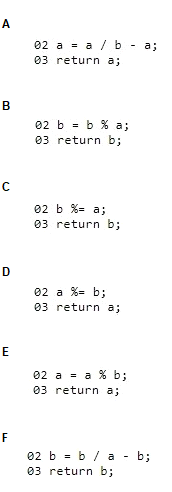# Microsoft 98-382 Introduction to Programming Using JavaScript Exam Practice Test

Page: 1 / 14
Total 39 questions

### Question 1

You are creating a web page that allows customers to choose how hot their spice is. If they choose spicy, a warning should be displayed.

You create the following form. Line numbers are included for reference only.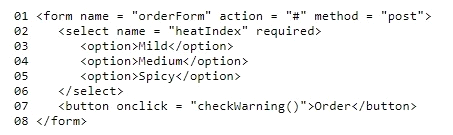You create the following JavaScript code to display the warning.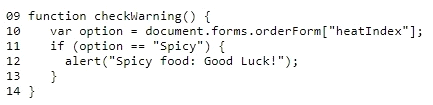When you choose spicy and click Order, the warning fails to display.

You need to solve this problem.

What should you do?

### Question 2

You are writing an engineering application. You need to create a function that will round numbers to 3 or more decimal places.

You need to create a function that receives the following two parameters:

The value parameter is the number to be formatted

The digits parameter is the number of digits to display

The function must return the value with the number of digits specified.

Which function should you use?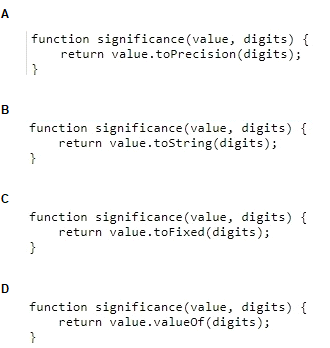### Question 3

This question requires that you evaluate the underlined text to determine if it is correct.

You review the following JavaScript code: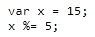When this code executes, the value of x is 0.

Review the underlined text. If it makes the statement correct, select ''No change is needed''. If the statement is incorrect, select the answer choice that makes the statement correct.

### Question 4

You are creating a JavaScript program for an accounting system.

You create the following code. Line numbers are included for reference only.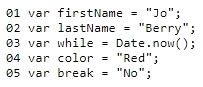You evaluate the code to ensure that it follows JavaScript best practices.

Which line should you change?

### Question 5

You are writing a function that calculates the remainder for integer division. The function receives two parameters, a and b, and must return the remainder that is left over when the integer a is divided by the integer b.

You create the following code. Line numbers are for reference only.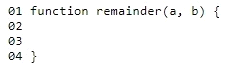You want to complete the function for lines 02 and 03.

Which two sets of code segments should you use? Each correct answer presents a complete solution. (Choose two.)